### Blinding Redness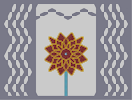Hover over the thumbnail for a full-size version.

Author Evil_Bob author:evil_bob n-art rated 2005-11-22 4 by 25 people. \$Blinding Redness#Evil_Bob#none#1111IF11111MIF111111IF111IG11HF11IG11HF11IG11HIG11IF11HG11IF11HG11IF111IG11HF11IG11HF11IG11HIG11IF11HG11IF11HG11IF111IG11HF11IG11HF11IG11HIG111111HG111111HG1111111111111111111111111111I00E000000000000000000F00C0000000000000000000000E000000000000000000000C0000000000000000000000B00000000000000000000000D0000000000000000000000B00000000000000000000000D0000000000000000000000E000000000000000000000C0000000000000000000000E000000000000000000000C0000000000000000000000B00000000000000000000000D00000000000000000000H0B0000000000000000000G11111111111111111111111IF111111IF111111IF1111111HF11IG11HF11IG11HF11IIF11HG11IF11HG11IF11HG111HF11IG11HF11IG11HF11IHF11HG11IF11HG11IF11HG111HF11IG11HF11IG11HF11I1111HG111111HG111111HG1|8^396,432,3!8^396,438,3!8^396,444,3!8^396,450,3!8^396,456,3!8^396,462,3!8^396,468,3!8^396,474,3!8^396,480,3!8^396,486,3!8^396,492,3!8^396,498,3!8^396,504,3!8^396,510,3!8^396,516,3!8^396,522,3!8^396,528,3!8^396,534,3!8^396,540,3!8^396,546,3!8^396,552,3!8^396,558,3!8^396,564,3!8^396,570,3!8^396,576,3!8^396,582,3!0^396,288!0^360,324!0^432,324!0^396,360!0^360,330!0^366,336!0^390,360!0^384,354!0^378,354!0^366,342!0^372,348!0^402,360!0^408,354!0^414,354!0^420,348!0^426,342!0^426,336!0^432,330!0^432,318!0^426,312!0^426,306!0^420,300!0^414,294!0^408,294!0^402,288!0^390,288!0^384,294!0^378,294!0^372,300!0^366,306!0^366,312!0^360,318!0^396,282!0^396,366!0^438,324!0^354,324!0^360,348!0^372,360!0^420,360!0^432,348!0^432,300!0^420,288!0^372,288!0^360,300!0^420,252!0^372,252!0^372,396!0^468,300!0^468,348!0^324,300!0^324,348!0^372,282!0^372,276!0^372,270!0^372,264!0^372,258!0^390,276!0^390,270!0^384,264!0^384,258!0^378,252!0^402,276!0^402,270!0^408,264!0^408,258!0^414,258!0^420,258!0^420,264!0^420,276!0^420,270!0^420,282!0^342,270!0^342,378!0^420,396!0^450,378!0^450,270!0^426,282!0^432,276!0^444,270!0^438,270!0^438,294!0^444,288!0^450,282!0^450,276!0^438,300!0^444,300!0^456,300!0^450,300!0^462,300!0^444,318!0^450,318!0^456,312!0^462,312!0^468,306!0^444,330!0^450,330!0^456,336!0^462,336!0^348,318!0^342,318!0^348,330!0^342,330!0^336,336!0^330,336!0^324,342!0^336,312!0^330,312!0^468,342!0^324,306!0^330,300!0^336,300!0^342,300!0^354,300!0^348,300!0^330,348!0^342,348!0^336,348!0^348,348!0^354,348!0^372,366!0^372,372!0^372,378!0^372,384!0^372,390!0^420,366!0^420,378!0^420,372!0^420,384!0^420,390!0^342,372!0^354,354!0^348,360!0^342,366!0^366,366!0^360,372!0^354,378!0^348,378!0^438,348!0^444,348!0^450,348!0^456,348!0^462,348!0^438,354!0^444,360!0^450,366!0^450,372!0^444,378!0^438,378!0^432,372!0^426,366!0^378,396!0^384,390!0^384,384!0^390,378!0^390,372!0^402,372!0^402,378!0^408,384!0^408,390!0^414,396!0^354,294!0^348,288!0^342,282!0^342,276!0^348,270!0^354,270!0^360,276!0^366,282!0^336,240!0^456,240!0^456,408!0^336,408!0^300,324!0^492,324!0^396,228!0^396,420!0^312,384!0^312,264!0^480,264!0^480,384!0^426,402!0^432,402!0^438,402!0^444,408!0^450,408!0^456,384!0^456,390!0^456,396!0^456,402!0^462,384!0^468,384!0^474,384!0^474,354!0^474,360!0^474,366!0^480,372!0^480,378!0^474,312!0^480,312!0^486,318!0^474,336!0^480,336!0^486,330!0^384,246!0^384,240!0^390,234!0^402,234!0^414,252!0^408,246!0^408,240!0^384,402!0^384,408!0^408,402!0^408,408!0^402,414!0^390,414!0^306,330!0^306,318!0^312,312!0^318,312!0^312,336!0^318,336!0^366,402!0^360,402!0^354,402!0^348,408!0^342,408!0^336,384!0^336,396!0^336,390!0^336,402!0^318,384!0^324,384!0^330,384!0^312,378!0^312,372!0^318,366!0^318,360!0^318,354!0^474,294!0^474,288!0^474,282!0^480,276!0^480,270!0^456,264!0^462,264!0^468,264!0^474,264!0^456,258!0^456,252!0^456,246!0^426,246!0^432,246!0^438,246!0^444,240!0^450,240!0^366,246!0^360,246!0^354,246!0^348,240!0^342,240!0^336,246!0^336,252!0^336,258!0^336,264!0^318,264!0^324,264!0^330,264!0^312,270!0^312,276!0^318,282!0^318,288!0^318,294!0^498,222!0^294,426!0^294,222!0^498,426!0^432,198!0^360,198!0^402,222!0^408,216!0^414,210!0^420,204!0^426,198!0^390,222!0^384,216!0^378,210!0^372,204!0^366,198!0^402,426!0^408,432!0^414,438!0^420,444!0^432,450!0^426,450!0^360,450!0^366,450!0^372,444!0^378,438!0^384,432!0^390,426!0^522,360!0^522,288!0^270,288!0^270,360!0^294,330!0^288,336!0^282,342!0^276,348!0^270,354!0^294,318!0^288,312!0^282,306!0^276,300!0^270,294!0^498,318!0^504,312!0^510,306!0^516,300!0^522,294!0^498,330!0^504,336!0^510,342!0^516,348!0^522,354!0^450,414!0^450,420!0^444,426!0^444,432!0^438,438!0^438,444!0^342,414!0^342,420!0^348,426!0^348,432!0^354,438!0^354,444!0^342,234!0^342,228!0^348,222!0^348,216!0^354,210!0^354,204!0^438,204!0^438,210!0^444,216!0^444,222!0^450,228!0^450,234!0^486,270!0^492,270!0^498,276!0^504,276!0^510,282!0^516,282!0^306,270!0^300,270!0^294,276!0^288,276!0^282,282!0^276,282!0^276,366!0^282,366!0^288,372!0^294,372!0^300,378!0^306,378!0^486,378!0^492,378!0^498,372!0^504,372!0^510,366!0^516,366!0^462,234!0^468,234!0^474,228!0^480,228!0^486,222!0^492,222!0^498,228!0^498,234!0^492,240!0^492,246!0^486,252!0^486,258!0^486,390!0^486,396!0^492,402!0^492,408!0^498,414!0^498,420!0^492,426!0^486,426!0^480,420!0^474,420!0^468,414!0^462,414!0^330,414!0^324,414!0^318,420!0^312,420!0^306,426!0^300,426!0^294,420!0^294,414!0^300,408!0^300,402!0^306,396!0^306,390!0^330,234!0^324,234!0^318,228!0^312,228!0^300,222!0^306,222!0^294,228!0^294,234!0^300,240!0^300,246!0^306,252!0^306,258!12^366,318!12^366,330!12^366,324!12^396,312!12^390,312!12^384,318!12^402,312!12^408,318!6^396,324,2,0,0,0!12^408,324!12^408,330!12^402,336!12^396,336!12^384,336!12^384,330!12^384,324!12^390,336!12^408,336!12^408,312!12^384,312!12^372,318!12^378,318!12^378,330!12^372,324!12^378,324!12^372,330!12^372,336!12^378,336!12^372,342!12^378,342!12^384,342!12^378,348!12^384,348!12^390,354!12^396,354!12^402,354!12^390,348!12^390,342!12^396,342!12^396,348!12^402,348!12^402,342!12^408,342!12^408,348!12^414,342!12^414,348!12^420,342!12^420,336!12^414,336!12^414,330!12^420,330!12^426,330!12^426,324!12^414,324!12^420,324!12^426,318!12^408,318!12^414,318!12^420,318!12^420,312!12^414,312!12^420,306!12^414,306!12^414,300!12^408,306!12^408,300!12^402,300!12^402,294!12^396,294!12^390,294!12^390,300!12^396,300!12^396,306!12^402,306!12^390,306!12^384,300!12^384,306!12^378,300!12^378,306!12^372,306!12^372,312!12^378,312!12^420,294!12^426,294!12^426,300!12^426,288!12^432,288!12^432,294!12^432,282!12^438,282!12^438,288!12^438,276!12^444,276!12^444,282!12^366,348!12^366,354!12^372,354!12^366,360!12^360,360!12^360,354!12^354,360!12^354,366!12^360,366!12^348,366!12^354,372!12^348,372!12^366,300!12^366,294!12^372,294!12^360,294!12^360,288!12^366,288!12^354,288!12^354,282!12^360,282!12^348,282!12^348,276!12^354,276!12^420,354!12^426,354!12^426,348!12^426,360!12^432,360!12^432,354!12^432,366!12^438,366!12^438,360!12^438,372!12^444,372!12^444,366!12^432,342!12^432,336!12^438,336!12^438,330!12^438,342!12^444,342!12^444,336!12^450,336!12^450,342!12^456,342!12^462,342!12^438,318!12^438,312!12^432,312!12^432,306!12^438,306!12^444,306!12^450,306!12^456,306!12^462,306!12^450,312!12^444,312!12^402,282!12^408,282!12^408,276!12^408,270!12^414,270!12^414,264!12^414,276!12^414,282!12^414,288!12^408,288!12^384,288!12^378,288!12^378,282!12^378,276!12^378,270!12^378,264!12^378,258!12^384,270!12^384,282!12^384,276!12^390,282!12^378,360!12^378,366!12^378,372!12^378,378!12^378,384!12^378,390!12^384,378!12^384,372!12^384,366!12^384,360!12^390,366!12^402,366!12^408,366!12^408,360!12^414,360!12^414,366!12^414,372!12^408,372!12^408,378!12^414,378!12^414,384!12^414,390!12^330,342!12^336,342!12^342,342!12^342,336!12^348,336!12^348,342!12^354,342!12^360,342!12^360,336!12^354,336!12^354,330!12^444,294!12^450,294!12^450,288!12^456,288!12^456,294!12^462,294!12^462,288!12^468,288!12^468,294!12^456,276!12^456,270!12^456,282!12^462,276!12^462,276!12^462,270!12^468,270!12^474,270!12^474,276!12^468,276!12^468,282!12^462,282!12^444,324!12^450,324!12^456,318!12^456,324!12^456,330!12^462,330!12^468,330!12^468,336!12^480,330!12^474,330!12^486,324!12^480,324!12^480,318!12^474,318!12^468,318!12^468,312!12^462,318!12^462,324!12^468,324!12^474,324!12^444,354!12^450,354!12^456,354!12^462,354!12^468,354!12^468,360!12^468,366!12^468,378!12^468,372!12^474,372!12^474,378!12^462,378!12^456,378!12^456,372!12^462,372!12^462,366!12^462,360!12^456,360!12^456,366!12^450,360!12^396,372!12^396,378!12^390,384!12^396,384!12^402,384!12^402,390!12^396,390!12^390,390!12^390,396!12^384,396!12^396,396!12^402,396!12^408,396!12^402,402!12^396,402!12^390,402!12^390,408!12^402,408!12^396,414!12^396,408!12^396,276!12^396,270!12^390,264!12^396,264!12^402,264!12^396,258!12^390,258!12^384,252!12^390,252!12^390,246!12^390,240!12^396,240!12^396,234!12^402,240!12^402,246!12^396,246!12^402,252!12^408,252!12^402,258!12^396,252!12^330,306!12^336,306!12^342,306!12^348,306!12^354,306!12^360,306!12^360,312!12^354,312!12^348,312!12^342,312!12^354,318!12^348,324!12^342,324!12^336,324!12^336,318!12^336,330!12^330,330!12^330,324!12^330,318!12^324,312!12^324,318!12^324,324!12^324,330!12^324,336!12^318,330!12^318,324!12^318,318!12^312,318!12^312,324!12^312,330!12^306,324!12^474,300!12^474,306!12^480,306!12^480,300!12^480,294!12^480,288!12^480,282!12^486,276!12^486,288!12^486,282!12^486,300!12^486,294!12^486,306!12^486,312!12^492,312!12^492,318!12^498,312!12^498,306!12^492,306!12^492,300!12^498,300!12^504,300!12^504,306!12^510,300!12^510,294!12^516,294!12^516,288!12^510,288!12^504,282!12^504,288!12^504,294!12^498,294!12^498,288!12^498,282!12^492,276!12^492,282!12^492,288!12^492,294!12^426,276!12^426,270!12^426,264!12^426,258!12^426,252!12^432,252!12^438,252!12^450,252!12^450,246!12^444,246!12^444,252!12^450,258!12^450,258!12^444,258!12^438,258!12^432,258!12^432,264!12^432,270!12^438,264!12^444,264!12^450,264!12^366,276!12^366,270!12^366,264!12^366,252!12^366,258!12^360,252!12^354,252!12^348,252!12^348,246!12^342,246!12^342,252!12^342,258!12^348,258!12^354,258!12^360,258!12^360,264!12^360,270!12^354,264!12^348,264!12^342,264!12^348,294!12^342,294!12^336,294!12^330,294!12^324,294!12^324,288!12^330,288!12^336,288!12^342,288!12^336,282!12^336,276!12^336,270!12^330,270!12^324,270!12^318,270!12^318,276!12^324,276!12^330,276!12^330,282!12^324,282!12^426,372!12^426,378!12^426,384!12^426,396!12^426,390!12^432,396!12^438,396!12^444,396!12^444,402!12^450,402!12^450,396!12^450,390!12^450,384!12^444,384!12^438,384!12^432,384!12^432,378!12^432,390!12^438,390!12^444,390!12^324,354!12^330,354!12^342,354!12^336,354!12^348,354!12^342,360!12^336,360!12^330,360!12^324,360!12^324,366!12^330,366!12^336,366!12^336,372!12^330,372!12^318,372!12^324,372!12^318,378!12^324,378!12^330,378!12^336,378!12^348,402!12^342,402!12^342,396!12^348,396!12^354,396!12^366,396!12^360,396!12^366,390!12^366,384!12^366,372!12^366,378!12^360,378!12^360,384!12^360,390!12^354,390!12^354,384!12^348,384!12^348,390!12^342,390!12^342,384!12^462,258!12^462,252!12^462,246!12^462,240!12^468,240!12^474,240!12^474,234!12^480,234!12^486,234!12^486,228!12^492,228!12^492,234!12^480,240!12^486,240!12^486,246!12^474,246!12^480,246!12^468,246!12^468,252!12^474,252!12^480,252!12^480,258!12^474,258!12^468,258!12^300,414!12^300,420!12^306,420!12^306,414!12^306,402!12^312,408!12^306,408!12^318,414!12^312,414!12^318,408!12^324,408!12^330,408!12^330,402!12^330,396!12^330,390!12^324,390!12^318,390!12^312,390!12^312,396!12^312,402!12^318,402!12^324,402!12^324,396!12^318,396!12^312,258!12^318,258!12^324,258!12^330,258!12^330,252!12^330,246!12^330,240!12^324,240!12^318,240!12^318,234!12^312,234!12^306,234!12^306,228!12^300,228!12^300,234!12^306,240!12^306,246!12^312,252!12^312,246!12^312,240!12^318,246!12^324,246!12^318,252!12^324,252!12^462,408!12^462,402!12^462,396!12^462,390!12^474,390!12^468,390!12^480,390!12^480,396!12^474,396!12^468,396!12^468,402!12^474,402!12^480,402!12^486,402!12^486,408!12^480,408!12^474,408!12^468,408!12^474,414!12^486,414!12^492,414!12^480,414!12^486,420!12^492,420!12^474,342!12^474,348!12^480,342!12^486,342!12^486,336!12^492,336!12^492,330!12^492,342!12^498,342!12^498,336!12^504,342!12^504,348!12^510,348!12^510,354!12^516,354!12^516,360!12^510,360!12^504,360!12^504,366!12^498,366!12^486,366!12^492,366!12^492,372!12^486,372!12^480,366!12^480,360!12^480,354!12^480,348!12^486,348!12^486,354!12^486,360!12^492,360!12^492,354!12^492,348!12^498,348!12^498,360!12^498,354!12^504,354!12^414,246!12^420,246!12^414,240!12^420,240!12^426,240!12^432,240!12^438,240!12^438,234!12^444,234!12^444,228!12^438,228!12^438,222!12^438,216!12^408,234!12^408,228!12^402,228!12^408,222!12^414,222!12^414,216!12^420,216!12^420,210!12^426,210!12^426,204!12^432,204!12^432,210!12^432,222!12^426,216!12^432,216!12^426,222!12^420,222!12^414,228!12^414,234!12^420,234!12^420,228!12^426,228!12^426,234!12^432,234!12^432,228!12^372,246!12^378,246!12^378,240!12^372,240!12^366,240!12^360,240!12^354,240!12^354,234!12^348,234!12^348,228!12^354,228!12^354,222!12^354,216!12^360,204!12^366,204!12^366,210!12^360,210!12^372,210!12^372,216!12^366,216!12^360,216!12^360,228!12^360,222!12^366,222!12^372,222!12^378,222!12^378,216!12^384,222!12^384,228!12^390,228!12^384,234!12^378,234!12^378,228!12^372,228!12^366,228!12^372,234!12^360,234!12^366,234!12^402,420!12^408,420!12^408,414!12^414,414!12^414,408!12^414,402!12^420,402!12^420,408!12^432,408!12^438,408!12^426,408!12^426,414!12^432,414!12^438,414!12^444,414!12^444,420!12^294,348!12^294,354!12^294,366!12^294,360!12^300,360!12^306,348!12^306,354!12^306,360!12^306,366!12^360,414!12^366,414!12^372,414!12^366,420!12^366,426!12^366,432!12^360,432!12^372,432!12^420,414!12^414,420!12^420,420!12^426,420!12^432,420!12^438,420!12^438,426!12^438,432!12^432,432!12^432,426!12^426,426!12^426,432!12^426,438!12^432,438!12^426,444!12^432,444!12^420,438!12^420,432!12^420,426!12^414,426!12^414,432!12^408,426!12^276,288!12^276,294!12^282,288!12^282,294!12^282,300!12^288,282!12^294,282!12^300,276!12^306,276!12^300,282!12^306,282!12^312,282!12^312,288!12^312,294!12^312,306!12^318,300!12^312,300!12^318,306!12^306,312!12^300,312!12^300,318!12^294,312!12^288,306!12^288,300!12^288,294!12^288,288!12^300,288!12^294,288!12^306,288!12^306,294!12^300,294!12^294,294!12^294,300!12^306,300!12^306,300!12^306,306!12^300,306!12^300,300!12^294,306!12^294,336!12^300,336!12^306,336!12^300,330!12^318,342!12^318,348!12^276,354!12^276,360!12^282,348!12^282,354!12^282,360!12^300,372!12^306,372!12^348,414!12^348,420!12^372,402!12^378,402!12^384,420!12^384,414!12^384,426!12^390,420!12^360,444!12^366,438!12^360,438!12^366,444!12^372,438!12^354,432!12^354,426!12^360,426!12^360,420!12^354,420!12^354,414!12^354,408!12^360,408!12^366,408!12^372,408!12^378,408!12^378,414!12^378,420!12^378,426!12^378,432!12^372,426!12^372,420!12^300,366!12^288,366!12^288,360!12^288,354!12^288,348!12^288,342!12^294,342!12^300,342!12^300,348!12^300,354!12^306,342!12^312,342!12^312,348!12^312,360!12^312,354!12^312,366!12^402,318!12^390,318!12^390,330!12^402,330# As you can prabably tell this took a bit of thime so please check it out. It is not exactly what I had in mind. I was hopeing to make more of a sequel to this http://numa.notdot.net/map/18232 (I guess it still is...) But instead of a cool fractal like thingy I got a cool flower like thingy...So behold the blinding Redness...

## Other maps by this author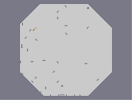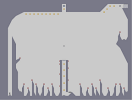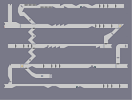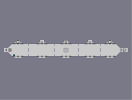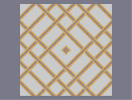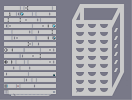Octogonal House of Horror Aichmophobia Unearthly Speeds The Never Ending Tube of Spite Fractal Thingy The Cheese Grater

Pages: (0)

ITS GOOD

### Best. one OF the BEST that IS...

wicked. 5/5 Dude, 5/5. Total 1337

### Templex:

You can't snap floorguards vertically, they automatically go to the bottom of the square. It wouldn't create the same effect that thwumps can :)

pretty

### Hmmm...

Well I thought of that but hey - What is done is done...
Uh.. Floorguards.

### It is the closest thing to green...

To bad you rated it down from a perfect 5 to 4.5 but it doesn't mattter...
Nice work. Why'd you use thwumps for the stem?